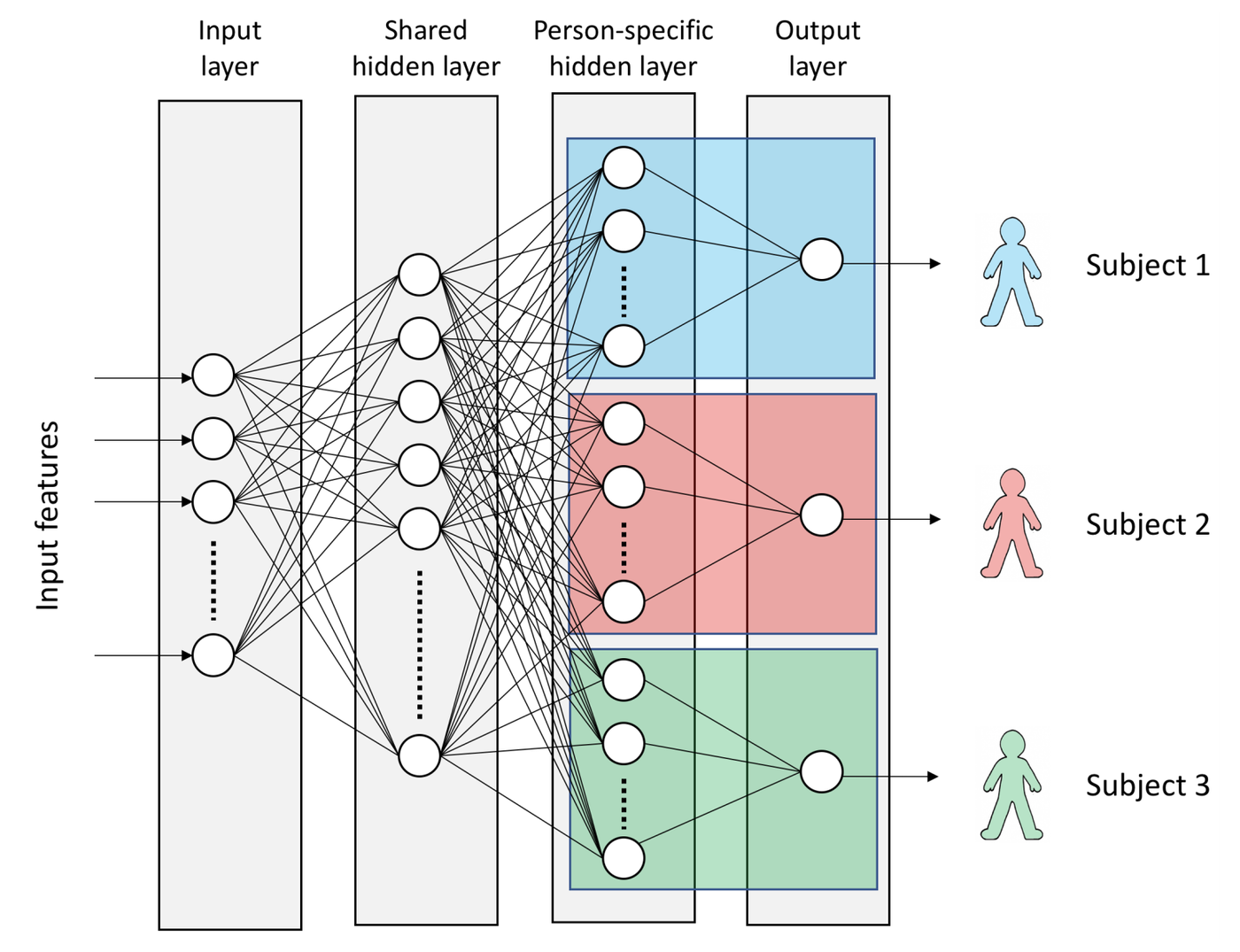# Multi task learning in Keras

I am trying to implement shared layers in Keras. I do see that Keras has keras.layers.concatenate, but I am unsure from documentation about its use. Can I use it to create multiple shared layers? What would be the best way to implement a simple shared neural network as shown below using Keras?Note that all the shape of input, output and shared layers for all 3 NNs are the same. There are multiple shared layers (and non-shared layers) in the three NNs. The coloured layers are unique to each NN, and have same shape.

Basically, the figure represents 3 identical NNs with multiple shared hidden layers, followed by multiple non-shared hidden layers.

I am unsure how to share multiple layers as in the Twitter example, there was just one shared layer (example in API doc).

By using the functional API you can easily share weights between different parts of your network. In your case we have an Input $x$ which is our input, then we will have a Dense layer called shared. Then we will have three different Dense layers called sub1, sub2 and sub3 and then three output layers called out1, out2 and out3.

x = Input(shape=(n, ))
shared = Dense(32)(x)
sub1 = Dense(16)(shared)
sub2 = Dense(16)(shared)
sub3 = Dense(16)(shared)
out1 = Dense(1)(sub1)
out2 = Dense(1)(sub2)
out3 = Dense(1)(sub3)


We can define our model now like this:

model = Model(inputs=x, outputs=[out1, out2, out3])


It will expect a tuple/list of three elements now, one for each output.

You can go a lot further with these concepts. Let's say we would like to learn individual weights for the person layers but still want to have the same weights for the linear combination towards the output layer, we could achieve that by doing this:

out = Dense(1)
out1 = out(sub1)
out2 = out(sub2)
out3 = out(sub3)


EDIT: Concatenating is basically the opposite of what you want to do, it's pasting (intermediate) outputs of different parts of your network into a new layer. You actually want to split off to multiple different parts.

• Thanks a lot. When we do a model.fit([data1, data2], [labels1, labels2]), this will be trained (the back propagation) as a single model, right? Feb 6 '18 at 21:59
• Yeah it will just be one thing, if the labels have different losses associated you will need to do some more work, it's not super easy in Keras but not impossible, if they share the same loss function without reweighting it just works out of the box Feb 6 '18 at 23:30
• This would require re-training the unified model. What if you already have the training weights for the sub-models? Is there a way to use those weights for creating the wieghts for the merged model? Jul 1 '18 at 7:24
• @shahar_m sorry I am unsure what the use case is. if the training weights of unified model are fixed, you can load and freeze those layers. Aug 1 '18 at 3:36